Feynman Diagram

Also found in: Dictionary, Wikipedia.

Feynman diagram

A pictorial representation of elementary particles and their interactions. Feynman diagrams show paths of particles in space and time as lines, and interactions between particles as points where the lines meet.

The illustration shows Feynman diagrams for electron-electron scattering. In each diagram, the straight lines represent space-time trajectories of noninteracting electrons, and the wavy lines represent photons, particles that transmit the electromagnetic interaction. External lines at the bottom of each diagram represent incoming particles (before the interactions), and lines at the top, outgoing particles (after the interactions). Interactions between photons and electrons occur at the vertices where photon lines meet electron lines. See Electron, Photon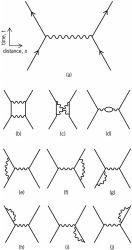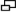Feynman diagrams for electron- electron (Møller) scattering: (a) second-order diagram (two- vertices); (b–j) fourth-order diagrams

Each Feynman diagram corresponds to the probability amplitude for the process depicted in the diagram. The set of all distinct Feynman diagrams with the same incoming and outgoing lines corresponds to the perturbation expansion of a matrix element of the scattering matrix in field theory. This correspondence can be used to formulate the rules for writing the amplitude associated with a particular diagram. The perturbation expansion and the associated Feynman diagrams are useful to the extent that the strength of the interaction is small, so that the lowest-order terms, or diagrams with the fewest vertices, give the main contribution to the matrix element. See Perturbation (quantum mechanics), Scattering matrix

Since their introduction in quantum electrodynamics, Feynman diagrams have been widely applied in other field theories. They are employed in studies of electroweak interactions, certain situations in quantum chromodynamics, in acoustooptics, and in many-body theory in atomic, nuclear, plasma, and condensed matter physics. See Acoustooptics, Elementary particle, Fundamental interactions, Quantum chromodynamics, Quantum electrodynamics, Quantum field theory, Weak nuclear interactions

Feynman Diagram

the basis of a graphical technique for the theoretical analysis of particle scattering and other physical processes and for the calculation of the amplitudes of the processes. The technique was proposed by R. Feynman in 1949 and played a very important part in the development of quantum electrodynamics. Feynman diagrams are widely used in quantum field theory, quantum mechanics, and statistical mechanics.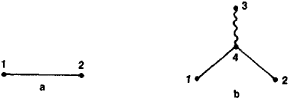Figure 1

A fundamental concept in Feynman’s diagrammatic technique is a propagation function called the Feynman propagator. The propagation of a wave field is equated with the motion of a particle in quantum theory, and—in accordance with Huygens’ principle—the wave field at every point in space and at every moment in time is regarded as a source of secondary waves. The Feynman propagator characterizes the propagation of a secondary wave between two space-time points. The propagator is a function of the two points (designated 1 and 2) and is represented by a line that joins the points (Figure l,a). The field at point 2 is given by the sum of the waves emitted from all possible points 1.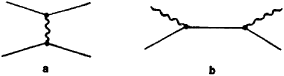Figure 2

In quantum theory, an interaction is regarded as the emission or absorption of waves or particles of various types. For example, the electromagnetic interaction results in the emission or absorption of an electromagnetic wave or a photon by an electron wave or an electron. The elementary event of such an interaction is depicted graphically by the diagram in Figure l,b, in which the straight lines are electron propagators and the wavy line is a photon propagator. The diagram indicates that when an electron wave propagates from point 1 to point 2, an electromagnetic field is emitted at point 4—the point of intersection of the lines, called the vertex of the diagram—and appears at point 3. By using the diagram in Figure l,b as a fundamental element, a Feynman diagram may be drawn for any electrodynamic process. For example, the diagram in Figure 2,a depicts the scattering of an electron by an electron or the collision of two electrons; the diagram in Figure 2,b depicts the scattering of a photon by an electron or the collision of a photon with an electron. In the diagrams, the external lines (the lines forming the vertices) represent the particles—an electron or a photon—before and after the collision or the scattering event, and the internal elements (the vertices and the wavy or solid line joining them) represent the interaction mechanism. In Figure 2,a the interaction results in the emission of an electromagnetic wave by the first electron and the absorption of the wave by the second electron. In Figure 2,b the interaction results in the emission of an electron wave. Thus, the motion of the respective particle in the virtual state corresponds to the propagation of a wave between the two vertices, that is, to an internal line. The same external line may represent either an initial particle or a final antiparticle, and vice versa. For example, the diagram in Figure 2,b, which should be viewed from the bottom to the top rather than from left to right, may represent the annihilation of an electron-positron pair and the resultant production of two photons.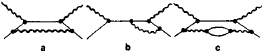Figure 3

The Feynman diagrams in Figure 2 correspond to the minimum number of elementary interactions, that is, to the minimum number of vertices that result in a given process. However, such interactions are not the only ones possible. A given type of particle collision is specified by the external lines, which represent the initial and final particles, respectively, but the internal part of a diagram may be more complex. For example, in addition to the diagram in Figure 2,b, the three Feynman diagrams shown in Figure 3, as well as many other diagrams, may be used to depict the scattering of a photon by an electron. In the diagrams in Figure 3 an electron, either incoming or virtual, emits a virtual photon, which is absorbed by the final electron. In Figure 3,c the virtual photon creates a virtual electron-positron pair that annihilates to produce a photon. If the magnitude of an interaction is small, the Feynman diagrams in Figure 3 and other diagrams containing a larger number of vertices—that is, a larger number of elementary interactions—yield only small corrections, called radiative corrections, in comparison with the contribution of the fundamental diagram in Figure 2,b. Thus, only a small number of diagrams is necessary if the magnitude of an interaction is small. This is also valid for quantum electrodynamics. In quantum electrodynamics, every additional internal line contributes a factor of e2/ℏc ≈ 1/137 to the scattering amplitude of the process considered; here, e is the charge of the electron, is Planck’s constant, and c is the speed of light. Hence, the predictions of quantum electrodynamics are highly accurate. However, if the magnitude of an interaction is not small, an infinite number of diagrams must be taken into account. This is a difficulty of quantum field theory.Figure 4

Feynman diagrams are also used to depict processes caused by other types of interactions. The decay of a neutral pion (π0) is shown in Figure 4, where the broken line is the π0, the solid lines are a nucleon and an antinucleon or a quark and an antiquark, the vertex on the left is the strong interaction, the wavy lines are photons, and the vertices formed on the right by the wavy lines are electromagnetic interactions. The decay of a charged pion (π+ or π) is shown in Figure 5; here, the broken line represents a π+ or a π, the lines forming the loop represent a nucleon and an antinucleon or a quark and an antiquark, the wavy line is a hypothetical vector boson (W+ or W, the carrier of the weak force), and the solid lines on the right represent a muon and a neutrino.Figure 5

Some factor corresponds to every element of a Feynman diagram, that is, to the incoming and outgoing lines, the vertices, and the internal lines. Therefore, if a Feynman diagram has been drawn, an analytical expression for the scattering amplitude of a given process may be immediately written.

REFERENCE

Schweber, S. Vvedenie v reliativistskuiu kvantovuiu teoriiu polia, ch. 14. Moscow, 1963. (Translated from English.)

V. B. BERESTETSKII

Feynman diagram

[′fīn·mən ‚dī·ə‚gram]
(quantum mechanics)
A diagram which gives an intuitive picture of a term in a perturbation expansion of a scattering matrix element or other physical quantity associated with interactions of particles; each line represents a particle, each vertex an interaction.
References in periodicals archive ?
Indeed, it is almost de rigueur to begin any treatment of Feynman diagrams by marveling at their ubiquity, and Adrian Wuthrich's The Genesis of Feynman Diagrams makes no exception.
Consider the Feynman diagram that looks a bit like a person in a spread-eagle stance.
Kaiser, David, Drawing Theories Apart: The Dispersion of Feynman Diagrams in Postwar Physics (Chicago: University of Chicago Press), 2005.
Richard Feynman, a brilliant physicist who gave his name in mathematics to the Feynman Diagrams, first presented his new theory of quantum electrodynamics at this 1948 meeting.
Physics is not just an aimless, random sequence of Feynman diagrams and symmetries, and mathematics is not just a set of messy equations, but rather physics and mathematics obey a definite symbiotic relationship.
Feynman's scientific work (his path-integral formulation of quantum mechanics, his contributions to quantum electrodynamics and the ubiquitous Feynman diagrams, his work on liquid helium and on the structure of nucleons) is also skillfully treated.
of Geneva) covers Lorentz and Poincare symmetries in quantum field theory, classical field theory, quantization of free fields, perturbation theory and Feynman diagrams, cross-section and decay rates, quantum electrodynamics, the low-energy limit of the electroweak theory, path integral quantization, non- abelian gauge theories, and spontaneous symmetry breaking.
The sidebar titled "Van Go" in the article on Richard Feynman states: "On May 11, 1998, Feynman diagrams were briefly in the public eye when a post office in Lake Worth, Fla.
In these proceedings from the conference of June 2001, participants describe their experience and research in developing graphs and patterns in such areas as Feynman diagrams (including in Hopf algebras and symmetries), algebraic structures (such as universal algebra, differential equations in noncommutative calculus, and twisted chiral de Rham algebras), manifolds, invariants and mirror symmetry (as in tri-level variants of three-folds, Massey products and the Johnson homomorphism), combinatorial aspects of dynamics (such as extensions, quotients and generalized pseudo-Anosov maps), and physics (such as in applications to study of black holes and the big bang).
was the beginning of a spotty but still-growing public awareness of Feynman diagrams, which first appeared in abstruse physics journals decades earlier.
Bar-Natan discovered simultaneously a remarkable link between his own work on Feynman diagrams - pictures used to provide an intuitive interpretation of interactions between subatomic particles - and Vassiliev's original equations for computing invariants.

Site: Follow: Share:
Open / Close